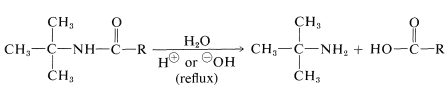# Making Amides from Nitriles

$$\newcommand{\vecs}{\overset { \rightharpoonup} {\mathbf{#1}} }$$ $$\newcommand{\vecd}{\overset{-\!-\!\rightharpoonup}{\vphantom{a}\smash {#1}}}$$$$\newcommand{\id}{\mathrm{id}}$$ $$\newcommand{\Span}{\mathrm{span}}$$ $$\newcommand{\kernel}{\mathrm{null}\,}$$ $$\newcommand{\range}{\mathrm{range}\,}$$ $$\newcommand{\RealPart}{\mathrm{Re}}$$ $$\newcommand{\ImaginaryPart}{\mathrm{Im}}$$ $$\newcommand{\Argument}{\mathrm{Arg}}$$ $$\newcommand{\norm}{\| #1 \|}$$ $$\newcommand{\inner}{\langle #1, #2 \rangle}$$ $$\newcommand{\Span}{\mathrm{span}}$$ $$\newcommand{\id}{\mathrm{id}}$$ $$\newcommand{\Span}{\mathrm{span}}$$ $$\newcommand{\kernel}{\mathrm{null}\,}$$ $$\newcommand{\range}{\mathrm{range}\,}$$ $$\newcommand{\RealPart}{\mathrm{Re}}$$ $$\newcommand{\ImaginaryPart}{\mathrm{Im}}$$ $$\newcommand{\Argument}{\mathrm{Arg}}$$ $$\newcommand{\norm}{\| #1 \|}$$ $$\newcommand{\inner}{\langle #1, #2 \rangle}$$ $$\newcommand{\Span}{\mathrm{span}}$$

The hydrolysis of nitriles is a satisfactory method for preparation of unsubstituted amides and is particularly convenient when hydrolysis is induced under mildly basic conditions by hydrogen peroxide:For the preparation of amides of the type $$\ce{R_3CNHCOR}$$, which have a tertiary alkyl group bonded to nitrogen, the Ritter reaction of an alcohol or alkene with a nitrile or hydrogen cyanide is highly advantageous. This reaction involves formation of a carbocation by action of strong sulfuric acid on an alkene or an alcohol (Equation 1), combination of the carbocation with the unshared electrons on nitrogen of $$\ce{RCN}$$ (Equation 2), and then addition of water (Equation 3). We use here the preparation of an $$\ce{N}$$-tert-butylalkanamide as an example; $$\ce{RC \equiv N}$$ can be an alkyl cyanide such as ethanenitrile or hydrogen cyanide itself:$$\tag{1}$$$$\tag{2}$$$$\tag{3}$$

This reaction also is useful for the preparation of primary amines by hydrolysis of the amide. It is one of the relatively few practical methods for synthesizing amines with a tertiary alkyl group on the nitrogen:## Contributors

John D. Robert and Marjorie C. Caserio (1977) Basic Principles of Organic Chemistry, second edition. W. A. Benjamin, Inc. , Menlo Park, CA. ISBN 0-8053-8329-8. This content is copyrighted under the following conditions, "You are granted permission for individual, educational, research and non-commercial reproduction, distribution, display and performance of this work in any format."

Making Amides from Nitriles is shared under a not declared license and was authored, remixed, and/or curated by LibreTexts.